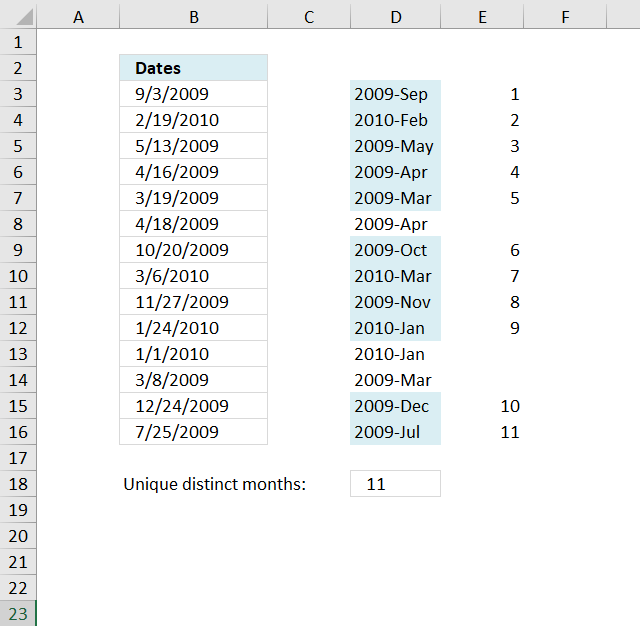Author: Oscar Cronquist Article last updated on November 29, 2018The formula in cell D18 counts unique distinct months in cell range B3:B16.

Formula in D18:

=SUMPRODUCT((FREQUENCY(DATE(YEAR(\$B\$3:\$B\$16), MONTH(\$B\$3:\$B\$16), 1), DATE(YEAR(\$B\$3:\$B\$16), MONTH(\$B\$3:\$B\$16), 1))>0)*1)

### Explaining formula in cell D18

#### Step 1 - Convert dates

The DATE function changes each date to the first in the given month and year.

DATE(YEAR(\$B\$3:\$B\$16), MONTH(\$B\$3:\$B\$16), 1)

becomes

DATE(YEAR({40059; 40228; 39946; 39919; 39891; 39921; 40106; 40243; 40144; 40202; 40179; 39880; 40171; 40019}), MONTH({40059; 40228; 39946; 39919; 39891; 39921; 40106; 40243; 40144; 40202; 40179; 39880; 40171; 40019}), 1)

becomes

DATE({2009; 2010; 2009; 2009; 2009; 2009; 2009; 2010; 2009; 2010; 2010; 2009; 2009; 2009}, {9; 2; 5; 4; 3; 4; 10; 3; 11; 1; 1; 3; 12; 7}, 1)

and returns

{40057; 40210; 39934; 39904; 39873; 39904; 40087; 40238; 40118; 40179; 40179; 39873; 40148; 39995}

#### Step 2 - Count frequency of each number

The FREQUENCY function returns an array of numbers representing how many times the number occurs in the list.

FREQUENCY(DATE(YEAR(\$B\$3:\$B\$16),MONTH(\$B\$3:\$B\$16),1),DATE(YEAR(\$B\$3:\$B\$16),MONTH(\$B\$3:\$B\$16),1))

becomes

FREQUENCY({40057; 40210; 39934; 39904; 39873; 39904; 40087; 40238; 40118; 40179; 40179; 39873; 40148; 39995},{40057; 40210; 39934; 39904; 39873; 39904; 40087; 40238; 40118; 40179; 40179; 39873; 40148; 39995})

and returns

{1;1;1;2;2;0;1;1;1;2;0;0;1;1;0}.

#### Step 3 - Check if value is larger than 0 (zero)

We know a value is unique distinct if it is larger than 0 (zero).

(FREQUENCY(DATE(YEAR(\$B\$3:\$B\$16),MONTH(\$B\$3:\$B\$16),1),DATE(YEAR(\$B\$3:\$B\$16),MONTH(\$B\$3:\$B\$16),1))>0

becomes

{1;1;1;2;2;0;1;1;1;2;0;0;1;1;0}>0

and returns

{TRUE; TRUE; TRUE; TRUE; TRUE; FALSE; TRUE; TRUE; TRUE; TRUE; FALSE; FALSE; TRUE; TRUE; FALSE}

#### Step 4 - Convert boolean values

We must first convert the boolean values in order to sum the values, multiply with 1 to create their numerical equivalents.

(FREQUENCY(DATE(YEAR(\$B\$3:\$B\$16), MONTH(\$B\$3:\$B\$16), 1), DATE(YEAR(\$B\$3:\$B\$16), MONTH(\$B\$3:\$B\$16), 1))>0)*1

becomes

{TRUE; TRUE; TRUE; TRUE; TRUE; FALSE; TRUE; TRUE; TRUE; TRUE; FALSE; FALSE; TRUE; TRUE; FALSE}*1

and returns

{1;1;1;1;1;0;1;1;1;1;0;0;1;1;0}.

#### Step 5 - Sum values

Use the SUMPRODUCT function to add the numbers and return a total.

SUMPRODUCT((FREQUENCY(DATE(YEAR(\$B\$3:\$B\$16),MONTH(\$B\$3:\$B\$16),1),DATE(YEAR(\$B\$3:\$B\$16),MONTH(\$B\$3:\$B\$16),1))>0)*1)

becomes

SUMPRODUCT({1;1;1;1;1;0;1;1;1;1;0;0;1;1;0})

and returns 11 in cell D18.

### Get Excel *.xlsx

Count unique distinct months.xlsx